telescopeѲptics.net          ▪▪▪▪                                             CONTENTS

# 8.1.3. Aberrations of the Newtonian reflector diagonal flat

The role of Newtonian diagonal flat mirror is to divert light converging from the primary to the side, so that the image forms outside the incoming light, and can be observed without obstructing it (except for the relatively small obstruction by the diagonal flat). Perfectly made diagonal flat doesn't induce optical aberrations; its only effects are: (1) usually small reduction in light transmission, equal to (1-ο2)r, with r being the diagonal reflectivity ratio, and ο its relative diameter in units of the aperture, and (2) worsening of the diffraction induced imaging error due to the effect of central obstruction.

Fast Newtonian telescopes usually have the flat "offset", or slightly shifted from the strictly centered position away from the focuser and toward the mirror, both directions by ∆=(D-V)/4[F-(1/16F)]D, in units of the diagonal flat's minor axis, where D and V are the aperture diameter and fully illuminated image field diameter, respectively, F-(1/16F) is the actual mirror focal ratio (when depth of sagitta is deducted from the focal length), and F is the conventional mirror focal ratio number F=f/D (offsetting amounts to sliding the surface downward along the inclination angle by 2∆). For all practical purposes, a simplified form ∆~(D-V)/4FD, or (D-V)/4f, in units of the minor axis (f being the mirror focal length), is just as accurate.

In order to distinguish this collimation mode from one in which diagonal moves only axially (unidirectional offset), it is referred to as bidirectional diagonal offset (BDO). Derivation of the relation for diagonal's bidirectional offset is given below the illustration on FIG. 117., as well as relations between the basic parameters.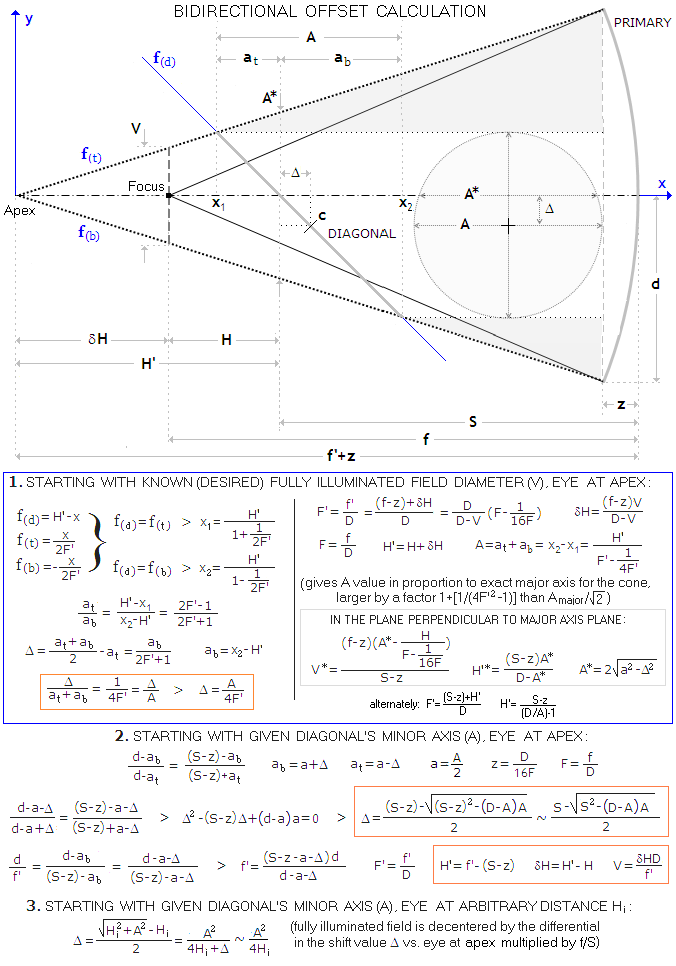FIGURE 117
: Needed diagonal bidirectional offset calculation is determined by the known parameters: it is either the primary and desired fully illuminated field, when needed diagonal size is also obtained (1), or the primary and diagonal size, with the resulting fully illuminated field (2). Shift value also changes with the eye-to-diagonal distance along the focuser axis (3). The geometry determining needed offset value is based on several parameters: mirror focal length
f, mirror-to-diagonal separation S, diagonal-to-focus separation H, diagonal-to-apex (apex being the point of convergence of the lines connecting top and bottom of the two mirrors) H', mirror sagitta z and fully illuminated field diameter V. Needed relations in (1) in are derived using three linear functions: for the diagonal surface, f(d), and for the top and bottom side of the extended cone, f(t) and f(b), respectively, in (2) from similar triangles (shaded gray), and in (3) from the identity of apparent angles over at and ab sections of the major axis.

The fully illuminated field diameter V depends on the flat's minor axis A and flat-to-focus separation H, as given by V=[(F-1/16F)A-H]/[(F-1/16F)-(H/D)]. Again, the difference is practically negligible when the relation is simplified to V~(FA-H)/[F-(H/D)]~[A-(H/F)]/[1-(H/f)]. Note that this gives fully illuminated field diameter in the horizontal plane, before the offset; with the diagonal offset, the field size in this plane is slightly reduced, due to the minor axis shift downward. Numerically negative result for V indicates too small flat size for its location. Substituting V into the above relation for the offset gives, after consolidation, more useable offset formula with all the factors known, ∆=(D-A)/4[(F-1/16F)D-H], in units of flat's minor axis A.

Replacing the actual mirror focal ratio F-(1/16F) with the conventional focal ratio number F doesn't make appreciable difference in the result. It leads to the final flat's offset formula - for eye at the apex point - as an actual shift away from the focuser and toward the mirror, as illustrated above: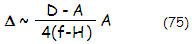If the diagonal-to-primary separation (f-H) is denoted as S, the relation simplifies to ∆~(D-A)A/4S.

Offsetting Newtonian diagonal flat doesn't influence image quality, but evens up field illumination and makes collimation easier, by centering the flat in the focuser opening (for near-minimum size diagonal flat, offsetting may also prevent a small light loss at the field center).

As the above illustration shows, this bidirectional offset value applies when the vantage point (location of the eye while collimating) is at the tip of the cone formed by extending lines connecting edges of primary and diagonal. For other vantage point heights, required bi-directional shift, derived from the equality of apparent angles of the two sections of diagonal's major axis on opposite sides of the focuser axis (identical in form to that preceding Eq. 74, for unidirectional offset) is also given by ∆=(Hi2+A2)/2, Hi being the vantage point height above the diagonal (fully illuminated field also becomes slightly decentered, with the decenter equaling the shift increase). This means that the diagonal offset in either bidirectional or unidirectional collimation mode is identical for given diagonal-to-eye separation prior to commencing the procedure

Most, if not all formulas, as well as online calculators, give the BDO shift value for collimating from the tip of extended cone, but that condition usually is not specified.

Minimum diagonal size (minor axis) to transmit the entire axial cone is given by Amin=H/F, H being the diagonal-to-focus separation (note that, due to the conical form of the converging cone, the major axis needs to be slightly larger than 2Amin, specifically [1+1/(4F2-1)]2Amin; this is however, of no significance in regular Newtonian systems). At the minimum secondary size, field illumination, normalized to 1 at the field center, is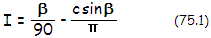with cos(β)=c/2 and c=[1-(H/f)]FV/H, where V is the image field diameter, f is the mirror focal length, and c is the axial center separation of the cone cross section at the diagonal, in units of diagonal's minor semi-axis. Quantitatively, c is determined by the overlap of the marginal ray of an off-axis point away from diagonal's edge in units of diagonal's minimum minor semi-axis. The actual nominal overlap value for the marginal ray is given by Cmin=[1-(H/f)]V/2 for the minimum diagonal size Amin, and for a larger diagonal A by CA=[(1-V/D)H/2F]-(A-V)/2.

Following graph (FIG. 118) shows illumination drop as a function of c, for a range of relative diagonal sizes.FIGURE 118: Illumination I of off-axis image points in the Newtonian as a function of the relative overlap of the marginal ray away from the diagonal's edge in units of the cone radius (c), for the actual minor axis A equal to the cone width at the diagonal (min. diagonal size), 1.5 and 2 times the minimum size. The illumination plot for the minimum size diagonal is identical to the MTF plot, since with c=2ν Eq. 75.1 is identical to Eq. 57. For c~1 and smaller, the plot changes linearly, with I=1-0.61c in that range of c values. Drop in illumination is somewhat slower for the larger minor axis A sizes, but not significantly. Linear diameter of the fully illuminated field is given by I100=[A-(H/F)]/[1-(H/f)]. The lowest acceptable limit to visual edge illumination is for c~1, with ~1 magnitude loss. The relative overlap ratio c in units of the minimum diagonal's semi-axis is given by c=1-[A-(1-H/f)V]F/H.

EXAMPLE: D=400mm f/4.5 Newtonian with diagonal-to-focus separation H=300mm, minimum diagonal minor axis Amin=66.7mm and actual minor axis A=80mm. Diameter of fully illuminated field is I100=16mm (0.5° angularly), relative overlap of marginal ray for (V/2)=22mm off-axis point is c=1-[A-(1-H/f)V]F/H=0.35, in units of the minimum diagonal's semi-axis. Corresponding illumination for the minimum diagonal size is I=(β/90)-csinβ/π=0.78; from FIG. 74, gain in illumination for A/Amin=1.2 for c=0.35 is nearly 2%, with the appropriate illumination at 22mm off-axis I~0.8, or nearly 80%.

As the plots show, field illumination drop-off with a minimum size diagonal is not significantly faster than with larger diagonal sizes. Even double the minimum size diagonal gains only about 10% illumination at most, for the field points with the converging cone overlapping the diagonal by about a length equal to the minimum diagonal size minor semi-axis (c~1). The real advantage of a larger diagonal is the size of fully illuminated field, which is zero for the minimum diagonal size, and increasing in proportion to diagonal size over the minimum size. Expressing the diagonal minor axis in units of aperture diameter D, as a, and diagonal to focus distance in units of the focal length, as h, the field of full illumination diameter is simply:

I100=(a-h)D/(1-h)          (75.2)

Evidently, for non-zero field of full illumination, relative size of diagonal's mirror axis in units of the aperture has to be larger than the relative size of diagonal-to-focus separation in units of the focal length. The size of fully illuminate field is strongly dependant on a-h and weakly (and inversely) dependant on 1-h. However, increase in h does not result in larger fully illuminated field with given diagonal, since it decreases a-h. For instance, increasing the diagonal-to-focuser separation from 0.15f to 0.2f automatically increases the minimum diagonal size by 0.05f/F=0.05D which, depending on the diagonal's size over the minimum size at h=0.15, will cause anything from significantly reducing field of full illumination to stopping the aperture down at the diagonal.

Mel Bartels' Newtonian diagonal calculator is a handy tool for obtaining field illumination figures in a specified Newtonian system. For computerized aid to this and other design aspects of a Newtonian, see Dave Keller's NEWT freeware.

Wavefront error at the diagonal is of particular interest with the Newtonian design, the diagonal flat being its constitutive element. Of course, perfectly flat diagonal won't induce any aberrations. In reality, every diagonal is less than perfectly flat, and the result is some form of wavefront deformation. Magnitude of this deformation depends directly on the size and type of surface error at the diagonal. In general, there are two types of diagonal surface error: (1) local error, covering relatively small portion of its surface, and (2) error smoothly distributed over the most, or all of the surface.

Any local surface error, be it a single defect, any number of local errors scattered over the surface, turned edge, or zone, will multiply in the wavefront by a factor of 2, or ~1.4. This is the consequence of its ~45° surface inclination, making the diagonal about 30% less sensitive to local surface errors and roughness than a "regular" mirror (FIG. 119).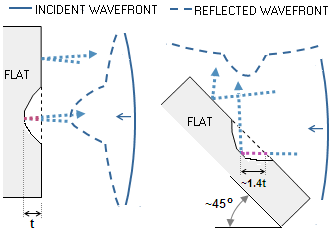FIGURE 119: Left: When the direction of light after reflection is nearly opposite to its incoming direction, local surface error resulting in an air path difference of the thickness t results in the wavefront P-V error of ~2t. This applies to both, flat and curved surface mirrors orthogonal to the optical axis.
Right: For the diagonal surface, the wavefront points after reflection move in nearly orthogonal direction to that of wavefront points coming onto the surface. Consequently, there is no added compounding of the surface error in the wavefront. A surface error creating an air path difference t will result in the wavefront P-V error of ~1.4t, due to the actual surface error (i.e. optical path difference) being enlarged by the ~45° position angle. Neither surface angles nor angles of convergence in amateur telescopes result in appreciable difference in added path difference vs. strict "orthogonal" scenario. In general, Newtonian diagonal flat is less sensitive to surface errors than main mirror.

For errors smoothly distributed over diagonal's surface it is somewhat more complicated. The reason is that they, in general, change the curvature of the wavefront so that part of the error induced can be compensated by refocusing. As long as radii depth along the minor and major axis are equal, there is no astigmatism induced, and the coma wavefront error is very small in comparison to the surface error. It is the difference in depth along the two radii that induces astigmatism, not the surface P-V error itself  (FIG. 120). Due to the inclination angle, the final P-V wavefront error of astigmatism is ~1.4 times the difference in radii depth (when the radii are of the opposite sign, as they are for the saddle surface form, the error is 1.4 times the sum of their depths). This conveniently allows for obtaining good approximations of the size of wavefront errors induced by these types of surface error using quite simple calculations.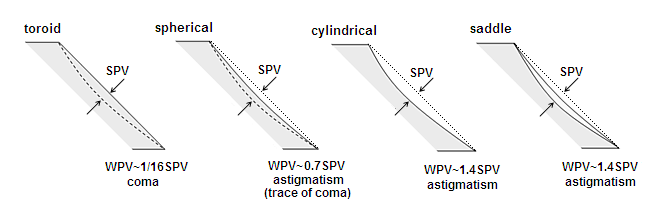FIGURE 120
: Four major types of smooth diagonal surface error, shown in the side view along the major axis. The toroid with the minor axis radius of curvature shorter by a factor of 2 than the major axis radius, has equal depths along both axes and flat edges, resulting in near-zero astigmatism and  P-V wavefront error (WPV) of coma ~1/16 of the surface P-V error (SPV). As the radii change toward equalization, depths along the two radii grow uneven, and astigmatism induced to the wavefront increases. Spherical surface has equal radii along both axes, resulting in uneven edge with the center depth along the minor axis being half of that along the major axis. The wavefront error induced - mostly astigmatism, with traces of coma - comes from the difference in radii depth, multiplied by a 2 factor, due to the 45° angle of surface inclination. Thus, the wavefront error is ~0.7 of the surface P-V error. Surface change toward cylindrical and saddle form result in further increase of the induced wavefront error of astigmatism: it  becomes equal to the surface error multiplied by ~1.4 factor (cylindrical form has the best focus RMS error lower nearly 20%). Concavity vs. convexity of the surface deformation is not a factor in the surface/wavefront P-V error relationship, and neither is the axis - major or minor - along which are oriented cylindrical and saddle form surface deformation. Also, the wavefront error is independent of focal ratio and aperture. Note that the diagonal surface error is for the area on the diagonal transmitting the wavefront, normally somewhat smaller than the entire diagonal. Since astigmatism changes with the square of aperture (i.e. beam diameter in this case), and coma with the third power of it, typical diagonal will have even smaller coma contributon.

The dependence of the size of wavefront error caused by the diagonal flat on both, size and form of the diagonal's surface error makes its actual performance level uncertain, even if the nominal surface RMS error is known (the P-V error is, as usual, pretty much meaningless). Safe policy is to go with the worst-case scenario, expecting the surface error to multiply in the wavefront by a factor of ~1.4. Since the dominant error for the above smooth surface deviation forms is astigmatism (except toroid, which is very unlikely to result from flat fabrication process, and the error it produces is insignificant), the corresponding RMS error is smaller than P-V error by a factor of 24. Table below quantifies approximate degradation factor for three surface P-V error values (wavefront errors are given in units of wavelength).

 FORM OF DIAGONAL'S SURFACE DEFORMATION P-V SURFACE ERROR P-V WAVEFRONT ERROR RMS WAVEFRONT STREHL DEGRADATION FACTOR SPHERICAL (RMS=PV/√24) 1/4 1/5.6 0.036 0.95 1/6 1/8.5 0.024 0.977 1/10 1/14 0.015 0.991 CYLINDRICAL/SADDLE (RMS=PV/√24) 1/4 1/2.8 0.073 0.82 1/6 1/4.2 0.049 0.91 1/10 1/7.1 0.028 0.97 ROUGHNESS (RMS~PV/3) 1/4 1/2.8 0.119 0.57 1/6 1/4.2 0.079 0.78 1/10 1/7.1 0.047 0.91 ZONAL (RMS~PV/3.3 for zones on the outer half of the radius) 1/4 1/2.8 0.108 0.63 1/6 1/4.2 0.051 0.90 1/10 1/7.1 0.043 0.93 EDGE 1/4 1/2.8 90% zone 0.064 0.85 95% zone 0.045 0.92 1/6 1/4.3 90% zone 0.041 0.93 95% zone 0.029 0.967 1/10 1/7.1 90% zone 0.025 0.975 95% zone 0.018 0.987 For minimum size diagonals similar to turned edge on main mirror; for larger relative sizes, not affecting mid field performance LOCAL Wavefront P-V larger by a factor of ~1.4 than surface P-V. Varies with the relative size of area affected, generally significantly smaller effect for given P-V error than P-V errors distributed over entire surface

Again, it is assumed that the P-V error is effective over the area covered by a reflected cone of light. This is not much of a factor with the last four deformation forms, but it can be significant with smooth errors over entire surface. In that case, the effective P-V error scales with the square of radius; if the P-V error is, for instance, 1/4 wave over the entire surface, it will be only half as large for converging cone that covers 70% of its diameter.

As noted before, the P-V error alone tells little about specific effect of the diagonal on image quality. For the same 1/4 P-V value, the Strehl degradation factor can be anywhere from 0.95 with spherical surface form (or even less with a local surface error), to 0.57 with surface roughness. With an excellent 0.95 Strehl primary, the former would result in a 0.90 optics Strehl, and the latter in decidedly substandard 0.54.

With star diagonals, converging cone uses much smaller portion of the optical surface. Thus, even much larger errors smoothly distributed over most of the surface have no appreciable effect on wavefront quality. However, local surface errors, especially in the mid-area, transmitting the light for the central portion of the field, can have significantly greater effect. Another difference is that star diagonal will scatter light for given small-scale surface roughness to much smaller radius than Newtonian diagonal, due to larger relative diameter of surface irregularities in the reflected wavefront.